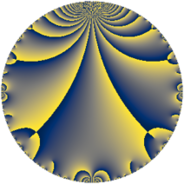# Properties

 Label 7.4.cLevel $7$ Weight $4$ Character orbit 7.c Rep. character $\chi_{7}(2,\cdot)$ Character field $\Q(\zeta_{3})$ Dimension $2$ Newform subspaces $1$ Sturm bound $2$ Trace bound $0$

# Related objects

## Defining parameters

 Level: $$N$$ $$=$$ $$7$$ Weight: $$k$$ $$=$$ $$4$$ Character orbit: $$[\chi]$$ $$=$$ 7.c (of order $$3$$ and degree $$2$$) Character conductor: $$\operatorname{cond}(\chi)$$ $$=$$ $$7$$ Character field: $$\Q(\zeta_{3})$$ Newform subspaces: $$1$$ Sturm bound: $$2$$ Trace bound: $$0$$

## Dimensions

The following table gives the dimensions of various subspaces of $$M_{4}(7, [\chi])$$.

Total New Old
Modular forms 6 6 0
Cusp forms 2 2 0
Eisenstein series 4 4 0

## Trace form

 $$2 q - 2 q^{2} - 7 q^{3} + 4 q^{4} - 7 q^{5} + 28 q^{6} + 28 q^{7} - 48 q^{8} - 22 q^{9} + O(q^{10})$$ $$2 q - 2 q^{2} - 7 q^{3} + 4 q^{4} - 7 q^{5} + 28 q^{6} + 28 q^{7} - 48 q^{8} - 22 q^{9} - 14 q^{10} + 5 q^{11} + 28 q^{12} - 28 q^{13} + 14 q^{14} + 98 q^{15} + 16 q^{16} + 21 q^{17} - 44 q^{18} - 49 q^{19} - 56 q^{20} - 245 q^{21} - 20 q^{22} + 159 q^{23} + 168 q^{24} + 76 q^{25} + 28 q^{26} - 70 q^{27} + 140 q^{28} + 116 q^{29} - 98 q^{30} - 147 q^{31} - 160 q^{32} + 35 q^{33} - 84 q^{34} + 49 q^{35} - 176 q^{36} - 219 q^{37} - 98 q^{38} + 98 q^{39} + 168 q^{40} + 700 q^{41} + 392 q^{42} - 248 q^{43} - 20 q^{44} - 154 q^{45} + 318 q^{46} - 525 q^{47} - 224 q^{48} + 98 q^{49} - 304 q^{50} + 147 q^{51} - 56 q^{52} - 303 q^{53} + 70 q^{54} - 70 q^{55} - 672 q^{56} + 686 q^{57} - 116 q^{58} + 105 q^{59} + 196 q^{60} + 413 q^{61} + 588 q^{62} + 154 q^{63} + 896 q^{64} + 98 q^{65} + 70 q^{66} - 415 q^{67} - 84 q^{68} - 2226 q^{69} - 490 q^{70} - 864 q^{71} + 528 q^{72} + 1113 q^{73} - 438 q^{74} + 532 q^{75} - 392 q^{76} + 175 q^{77} - 392 q^{78} + 103 q^{79} + 112 q^{80} + 839 q^{81} - 700 q^{82} + 2184 q^{83} - 196 q^{84} - 294 q^{85} + 248 q^{86} - 406 q^{87} - 120 q^{88} + 329 q^{89} + 616 q^{90} - 392 q^{91} + 1272 q^{92} - 1029 q^{93} - 1050 q^{94} - 343 q^{95} - 1120 q^{96} - 1764 q^{97} + 1078 q^{98} - 220 q^{99} + O(q^{100})$$

## Decomposition of $$S_{4}^{\mathrm{new}}(7, [\chi])$$ into newform subspaces

Label Dim $A$ Field CM Traces $q$-expansion
$a_{2}$ $a_{3}$ $a_{5}$ $a_{7}$
7.4.c.a $2$ $0.413$ $$\Q(\sqrt{-3})$$ None $$-2$$ $$-7$$ $$-7$$ $$28$$ $$q+(-2+2\zeta_{6})q^{2}-7\zeta_{6}q^{3}+4\zeta_{6}q^{4}+\cdots$$